# Double Factorials

in StemSocial10 days ago

Hi there. In this short math post, I cover the topic of double factorials.

Math text, symbols rendered with LaTeX with Quicklatex.com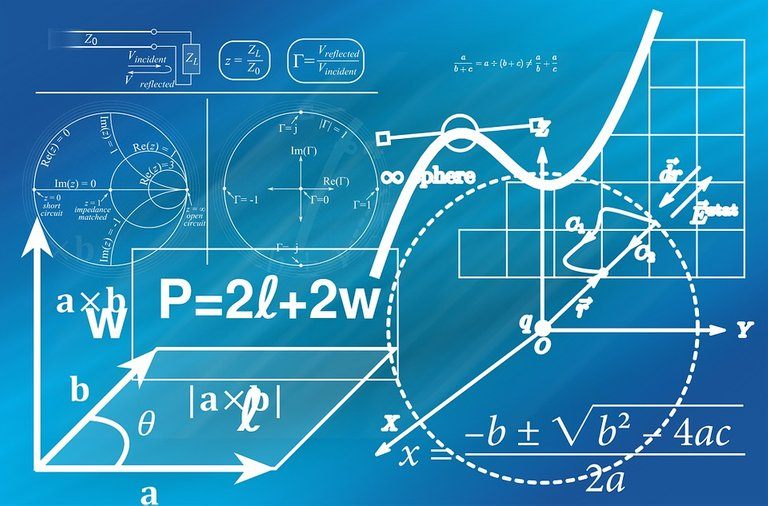Pixabay Image Source

## Topics

• Factorials
• Double Factorials
• Examples

## Factorials

A factorial is a compact way to express a number in the form of multiplying numbers together. The symbol associated with a factorial is the exclamation mark (!). As an example, 3! (three factorial) is `3 x 2 x 1 = 6`. The exclamation mark is a bit unusual. It does not mean that the number is shouting at you.

In general, the definition of a factorial is: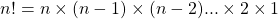for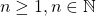(n is a positive whole number at least 1).

Zero Factorial Case

When it comes to factorials, zero factorial is not zero. It is actually equal to 1. `0! = 1`.

Expressing A Factorial With The Product Pi Notation

Another compact notation for factorials is the product pi notation. The product pi notation starts at 1 for the index variable `i` and increases to all the way to `n` in the product.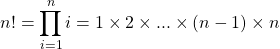Factorial Piecewise Function

A piecewise function can be developed around the factorial.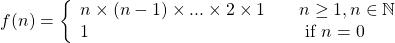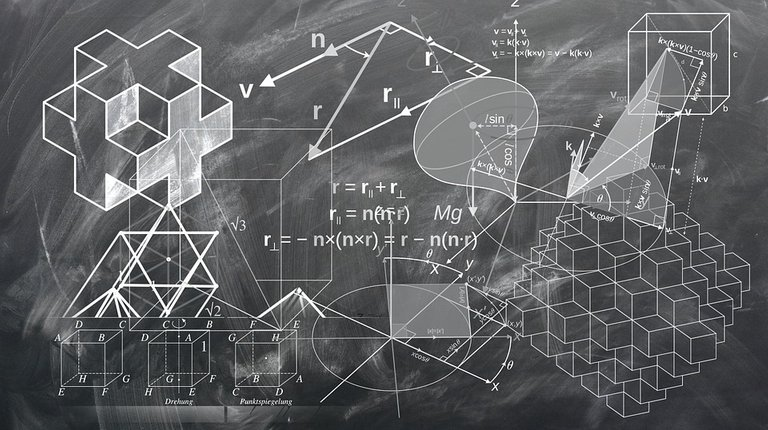Pixabay Image Source

## Double Factorials

A double factorial is symbolized by two exclamation marks. As a single factorial has numbers being spaced by 1, a double factorial contains numbers being spaced by two. The double factorial has two versions as the number `n` can be either odd or even.

If `n` is odd: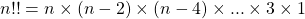For `n` being even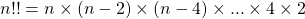With the double factorial, you may have the zero case and the negative one case. In either case they are equal to 1 as defined. That is `0!! = 1` and `-1!! = 1`.

Combining all these cases, a piecewise function can be developed with the three cases.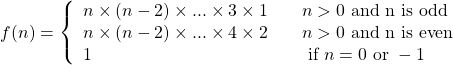Pixabay Image Source

## Examples

Example One

Having 3!! would be `3!! = 3 x 1 = 3`.

Example Two

What is the value of `10!!`?

`10!! = 10 x 8 x 6 x 4 x 2 = 3840`

Example Three

Divide 7!! by 7!.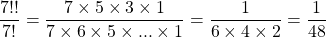Example Four

From example three, you can see that the double factorial is less than the single factorial given the same integer number `n`. What would be `n!` divided by `n!!`?

Use the definition of the factorials here, simplify and obtain the answer. This is done for the even and odd cases.

For `n > 0` being even: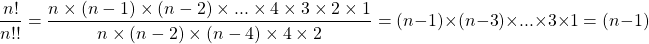With the `n > 0` being odd case, we have: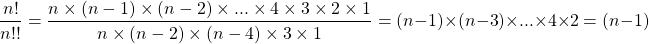In either case for `n`, the result would be: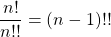Pixabay Image Source